# Algebra 1 Honors (#1200320)

This document was generated on CPALMS - www.cpalms.org
You are not viewing the current course, please click the current year’s tab.

#### Course Standards

Name Description
MA.912.AR.1.1: Identify and interpret parts of an equation or expression that represent a quantity in terms of a mathematical or real-world context, including viewing one or more of its parts as a single entity.
 Clarifications:Clarification 1: Parts of an expression include factors, terms, constants, coefficients and variables. Clarification 2: Within the Mathematics for Data and Financial Literacy course, problem types focus on money and business.

 Examples:Algebra 1 Example: Derrick is using the formula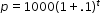to make a prediction about the camel population in Australia. He identifies the growth factor as (1+.1), or 1.1, and states that the camel population will grow at an annual rate of 10% per year.Example: The expression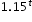can be rewritten as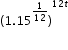which is approximately equivalent to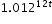. This latter expression reveals the approximate equivalent monthly interest rate of 1.2% if the annual rate is 15%.
MA.912.AR.1.2: Rearrange equations or formulas to isolate a quantity of interest.
 Clarifications:Clarification 1: Instruction includes using formulas for temperature, perimeter, area and volume; using equations for linear (standard, slope-intercept and point-slope forms) and quadratic (standard, factored and vertex forms) functions. Clarification 2: Within the Mathematics for Data and Financial Literacy course, problem types focus on money and business.

 Examples:Algebra 1 Example: The Ideal Gas Law PV = nRT can be rearranged as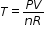to isolate temperature as the quantity of interest. Example: Given the Compound Interest formula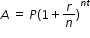, solve for P. Mathematics for Data and Financial Literacy Honors Example: Given the Compound Interest formula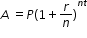, solve for t.
MA.912.AR.1.3: Add, subtract and multiply polynomial expressions with rational number coefficients.
 Clarifications:Clarification 1: Instruction includes an understanding that when any of these operations are performed with polynomials the result is also a polynomial.Clarification 2: Within the Algebra 1 course, polynomial expressions are limited to 3 or fewer terms.
MA.912.AR.1.4: Divide a polynomial expression by a monomial expression with rational number coefficients.
 Clarifications:Clarification 1: Within the Algebra 1 course, polynomial expressions are limited to 3 or fewer terms.
MA.912.AR.1.7: Rewrite a polynomial expression as a product of polynomials over the real number system.
 Clarifications:Clarification 1: Within the Algebra 1 course, polynomial expressions are limited to 4 or fewer terms with integer coefficients.

 Examples:Example: The expression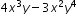is equivalent to the factored form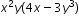. Example: The expression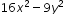is equivalent to the factored form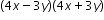.
MA.912.AR.2.1: Given a real-world context, write and solve one-variable multi-step linear equations.
MA.912.AR.2.2: Write a linear two-variable equation to represent the relationship between two quantities from a graph, a written description or a table of values within a mathematical or real-world context.
 Clarifications:Clarification 1: Instruction includes the use of standard form, slope-intercept form and point-slope form, and the conversion between these forms.
MA.912.AR.2.3: Write a linear two-variable equation for a line that is parallel or perpendicular to a given line and goes through a given point.
 Clarifications:Clarification 1: Instruction focuses on recognizing that perpendicular lines have slopes that when multiplied result in -1 and that parallel lines have slopes that are the same. Clarification 2: Instruction includes representing a line with a pair of points on the coordinate plane or with an equation. Clarification 3: Problems include cases where one variable has a coefficient of zero.
MA.912.AR.2.4: Given a table, equation or written description of a linear function, graph that function, and determine and interpret its key features.
 Clarifications:Clarification 1: Key features are limited to domain, range, intercepts and rate of change. Clarification 2: Instruction includes the use of standard form, slope-intercept form and point-slope form.Clarification 3: Instruction includes cases where one variable has a coefficient of zero.Clarification 4: Instruction includes representing the domain and range with inequality notation, interval notation or set-builder notation.Clarification 5: Within the Algebra 1 course, notations for domain and range are limited to inequality and set-builder notations.
MA.912.AR.2.5: Solve and graph mathematical and real-world problems that are modeled with linear functions. Interpret key features and determine constraints in terms of the context.
 Clarifications:Clarification 1: Key features are limited to domain, range, intercepts and rate of change.Clarification 2: Instruction includes the use of standard form, slope-intercept form and point-slope form.Clarification 3: Instruction includes representing the domain, range and constraints with inequality notation, interval notation or set-builder notation.Clarification 4: Within the Algebra 1 course, notations for domain, range and constraints are limited to inequality and set-builder.Clarification 5: Within the Mathematics for Data and Financial Literacy course, problem types focus on money and business.

 Examples:Algebra 1 Example: Lizzy’s mother uses the function C(p)=450+7.75p, where C(p) represents the total cost of a rental space and p is the number of people attending, to help budget Lizzy’s 16th birthday party. Lizzy’s mom wants to spend no more than \$850 for the party. Graph the function in terms of the context.
MA.912.AR.2.6: Given a mathematical or real-world context, write and solve one-variable linear inequalities, including compound inequalities. Represent solutions algebraically or graphically.
 Examples:Algebra 1 Example: The compound inequality 2x≤5x+1<4 is equivalent to -1≤3x and 5x<3, which is equivalent to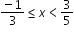.
MA.912.AR.2.7: Write two-variable linear inequalities to represent relationships between quantities from a graph or a written description within a mathematical or real-world context.
 Clarifications:Clarification 1: Instruction includes the use of standard form, slope-intercept form and point-slope form and any inequality symbol can be represented. Clarification 2: Instruction includes cases where one variable has a coefficient of zero.
MA.912.AR.2.8: Given a mathematical or real-world context, graph the solution set to a two-variable linear inequality.
 Clarifications:Clarification 1: Instruction includes the use of standard form, slope-intercept form and point-slope form and any inequality symbol can be represented. Clarification 2: Instruction includes cases where one variable has a coefficient of zero.
MA.912.AR.3.1: Given a mathematical or real-world context, write and solve one-variable quadratic equations over the real number system.
 Clarifications:Clarification 1: Within the Algebra 1 course, instruction includes the concept of non-real answers, without determining non-real solutions. Clarification 2: Within this benchmark, the expectation is to solve by factoring techniques, taking square roots, the quadratic formula and completing the square.
MA.912.AR.3.4: Write a quadratic function to represent the relationship between two quantities from a graph, a written description or a table of values within a mathematical or real-world context.
 Clarifications:Clarification 1: Within the Algebra 1 course, a graph, written description or table of values must include the vertex and two points that are equidistant from the vertex.Clarification 2: Instruction includes the use of standard form, factored form and vertex form.Clarification 3: Within the Algebra 2 course, one of the given points must be the vertex or an x-intercept.

Examples:
Algebra I Example: Given the table of values below from a quadratic function, write an equation of that function.
 x -2 -1 0 1 2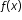2 -1 -2 -1 2
MA.912.AR.3.5: Given the x-intercepts and another point on the graph of a quadratic function, write the equation for the function.
MA.912.AR.3.6: Given an expression or equation representing a quadratic function, determine the vertex and zeros and interpret them in terms of a real-world context.
MA.912.AR.3.7: Given a table, equation or written description of a quadratic function, graph that function, and determine and interpret its key features.
 Clarifications:Clarification 1: Key features are limited to domain; range; intercepts; intervals where the function is increasing, decreasing, positive or negative; end behavior; vertex; and symmetry.Clarification 2: Instruction includes the use of standard form, factored form and vertex form, and sketching a graph using the zeros and vertex.Clarification 3: Instruction includes representing the domain and range with inequality notation, interval notation or set-builder notation.Clarification 4: Within the Algebra 1 course, notations for domain and range are limited to inequality and set-builder.
MA.912.AR.3.8: Solve and graph mathematical and real-world problems that are modeled with quadratic functions. Interpret key features and determine constraints in terms of the context.
 Clarifications:Clarification 1: Key features are limited to domain; range; intercepts; intervals where the function is increasing, decreasing, positive or negative; end behavior; vertex; and symmetry.Clarification 2: Instruction includes the use of standard form, factored form and vertex form.Clarification 3: Instruction includes representing the domain, range and constraints with inequality notation, interval notation or set-builder notation.Clarification 4: Within the Algebra 1 course, notations for domain, range and constraints are limited to inequality and set-builder.

 Examples:Algebra 1 Example: The value of a classic car produced in 1972 can be modeled by the function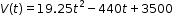, where t is the number of years since 1972. In what year does the car’s value start to increase?
MA.912.AR.4.1: Given a mathematical or real-world context, write and solve one-variable absolute value equations.
MA.912.AR.4.2: Given a mathematical or real-world context, write and solve one-variable absolute value inequalities. Represent solutions algebraically or graphically.
MA.912.AR.4.3: Given a table, equation or written description of an absolute value function, graph that function and determine its key features.
 Clarifications:Clarification 1: Key features are limited to domain; range; intercepts; intervals where the function is increasing, decreasing, positive or negative; vertex; end behavior and symmetry. Clarification 2: Instruction includes representing the domain and range with inequality notation, interval notation or set-builder notation.Clarification 3: Within the Algebra 1 course, notations for domain and range are limited to inequality and set-builder.
MA.912.AR.5.3: Given a mathematical or real-world context, classify an exponential function as representing growth or decay.
 Clarifications:Clarification 1: Within the Algebra 1 course, exponential functions are limited to the forms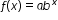, where b is a whole number greater than 1 or a unit fraction, or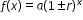, where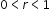.
MA.912.AR.5.4: Write an exponential function to represent a relationship between two quantities from a graph, a written description or a table of values within a mathematical or real-world context.
 Clarifications:Clarification 1: Within the Algebra 1 course, exponential functions are limited to the forms, where b is a whole number greater than 1 or a unit fraction, or, where.Clarification 2: Within the Algebra 1 course, tables are limited to having successive nonnegative integer inputs so that the function may be determined by finding ratios between successive outputs.
MA.912.AR.5.6: Given a table, equation or written description of an exponential function, graph that function and determine its key features.
 Clarifications:Clarification 1: Key features are limited to domain; range; intercepts; intervals where the function is increasing, decreasing, positive or negative; constant percent rate of change; end behavior and asymptotes. Clarification 2: Instruction includes representing the domain and range with inequality notation, interval notation or set-builder notation. Clarification 3: Within the Algebra 1 course, notations for domain and range are limited to inequality and set-builder. Clarification 4: Within the Algebra 1 course, exponential functions are limited to the forms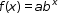, where b is a whole number greater than 1 or a unit fraction or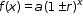, where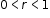.
MA.912.AR.9.1: Given a mathematical or real-world context, write and solve a system of two-variable linear equations algebraically or graphically.
 Clarifications:Clarification 1: Within this benchmark, the expectation is to solve systems using elimination, substitution and graphing.Clarification 2: Within the Algebra 1 course, the system is limited to two equations.
MA.912.AR.9.4: Graph the solution set of a system of two-variable linear inequalities.
 Clarifications:Clarification 1: Instruction includes cases where one variable has a coefficient of zero. Clarification 2: Within the Algebra 1 course, the system is limited to two inequalities.
MA.912.AR.9.6: Given a real-world context, represent constraints as systems of linear equations or inequalities. Interpret solutions to problems as viable or non-viable options.
 Clarifications:Clarification 1: Instruction focuses on analyzing a given function that models a real-world situation and writing constraints that are represented as linear equations or linear inequalities.
MA.912.DP.1.1: Given a set of data, select an appropriate method to represent the data, depending on whether it is numerical or categorical data and on whether it is univariate or bivariate.
 Clarifications:Clarification 1: Instruction includes discussions regarding the strengths and weaknesses of each data display. Clarification 2: Numerical univariate includes histograms, stem-and-leaf plots, box plots and line plots; numerical bivariate includes scatter plots and line graphs; categorical univariate includes bar charts, circle graphs, line plots, frequency tables and relative frequency tables; and categorical bivariate includes segmented bar charts, joint frequency tables and joint relative frequency tables. Clarification 3: Instruction includes the use of appropriate units and labels and, where appropriate, using technology to create data displays.
MA.912.DP.1.2: Interpret data distributions represented in various ways. State whether the data is numerical or categorical, whether it is univariate or bivariate and interpret the different components and quantities in the display.
 Clarifications:Clarification 1: Within the Probability and Statistics course, instruction includes the use of spreadsheets and technology.
MA.912.DP.1.3: Explain the difference between correlation and causation in the contexts of both numerical and categorical data.
 Examples:Algebra 1 Example: There is a strong positive correlation between the number of Nobel prizes won by country and the per capita chocolate consumption by country. Does this mean that increased chocolate consumption in America will increase the United States of America’s chances of a Nobel prize winner?
MA.912.DP.1.4: Estimate a population total, mean or percentage using data from a sample survey; develop a margin of error through the use of simulation.
 Clarifications:Clarification 1: Within the Algebra 1 course, the margin of error will be given.

 Examples:Algebra 1 Example: Based on a survey of 100 households in Twin Lakes, the newspaper reports that the average number of televisions per household is 3.5 with a margin of error of ±0.6. The actual population mean can be estimated to be between 2.9 and 4.1 television per household. Since there are 5,500 households in Twin Lakes the estimated number of televisions is between 15,950 and 22,550.
MA.912.DP.2.4: Fit a linear function to bivariate numerical data that suggests a linear association and interpret the slope and y-intercept of the model. Use the model to solve real-world problems in terms of the context of the data.
 Clarifications:Clarification 1: Instruction includes fitting a linear function both informally and formally with the use of technology.Clarification 2: Problems include making a prediction or extrapolation, inside and outside the range of the data, based on the equation of the line of fit.
MA.912.DP.2.5: Given a scatter plot that represents bivariate numerical data, assess the fit of a given linear function by plotting and analyzing residuals.
 Clarifications:Clarification 1: Within the Algebra 1 course, instruction includes determining the number of positive and negative residuals; the largest and smallest residuals; and the connection between outliers in the data set and the corresponding residuals.
MA.912.DP.2.6: Given a scatter plot with a line of fit and residuals, determine the strength and direction of the correlation. Interpret strength and direction within a real-world context.
 Clarifications:Clarification 1: Instruction focuses on determining the direction by analyzing the slope and informally determining the strength by analyzing the residuals.
MA.912.DP.3.1: Construct a two-way frequency table summarizing bivariate categorical data. Interpret joint and marginal frequencies and determine possible associations in terms of a real-world context.
Examples:
Algebra 1 Example: Complete the frequency table below.

 Has an A in math Doesn't have an A in math Total Plays an instrument 20 90 Doesn't play an instrument 20 Total 350

Using the information in the table, it is possible to determine that the second column contains the numbers 70 and 240. This means that there are 70 students who play an instrument but do not have an A in math and the total number of students who play an instrument is 90. The ratio of the joint frequencies in the first column is 1 to 1 and the ratio in the second column is 7 to 24, indicating a strong positive association between playing an instrument and getting an A in math.

MA.912.DP.3.2: Given marginal and conditional relative frequencies, construct a two-way relative frequency table summarizing categorical bivariate data.
 Clarifications:Clarification 1: Construction includes cases where not all frequencies are given but enough are provided to be able to construct a two-way relative frequency table. Clarification 2: Instruction includes the use of a tree diagram when calculating relative frequencies to construct tables.

Examples:
Algebra 1 Example: A study shows that 9% of the population have diabetes and 91% do not. The study also shows that 95% of the people who do not have diabetes, test negative on a diabetes test while 80% who do have diabetes, test positive. Based on the given information, the following relative frequency table can be constructed.
 Positive Negative Total Has diabetes 7.2% 1.8% 9% Doesn't have diabetes 4.55% 86.45% 91%

MA.912.DP.3.3: Given a two-way relative frequency table or segmented bar graph summarizing categorical bivariate data, interpret joint, marginal and conditional relative frequencies in terms of a real-world context.
 Clarifications:Clarification 1: Instruction includes problems involving false positive and false negatives.

Examples:
Algebra 1 Example: Given the relative frequency table below, the ratio of true positives to false positives can be determined as 7.2 to 4.55, which is about 3 to 2, meaning that a randomly selected person who tests positive for diabetes is about 50% more likely to have diabetes than not have it.

 Positive Negative Total Has diabetes 7.2% 1.8% 9% Doesn't have diabetes 4.55% 86.45% 91%

MA.912.F.1.1: Given an equation or graph that defines a function, determine the function type. Given an input-output table, determine a function type that could represent it.
 Clarifications:Clarification 1: Within the Algebra 1 course, functions represented as tables are limited to linear, quadratic and exponential. Clarification 2: Within the Algebra 1 course, functions represented as equations or graphs are limited to vertical or horizontal translations or reflections over the x-axis of the following parent functions: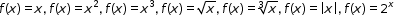and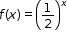.
MA.912.F.1.2: Given a function represented in function notation, evaluate the function for an input in its domain. For a real-world context, interpret the output.
 Clarifications:Clarification 1: Problems include simple functions in two-variables, such as f(x,y)=3x-2y. Clarification 2: Within the Algebra 1 course, functions are limited to one-variable such as f(x)=3x.

 Examples:Algebra 1 Example: The function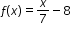models Alicia’s position in miles relative to a water stand x minutes into a marathon. Evaluate and interpret for a quarter of an hour into the race.
MA.912.F.1.3: Calculate and interpret the average rate of change of a real-world situation represented graphically, algebraically or in a table over a specified interval.
 Clarifications:Clarification 1: Instruction includes making the connection to determining the slope of a particular line segment.
MA.912.F.1.5: Compare key features of linear functions each represented algebraically, graphically, in tables or written descriptions.
 Clarifications:Clarification 1: Key features are limited to domain; range; intercepts; slope and end behavior.
MA.912.F.1.6: Compare key features of linear and nonlinear functions each represented algebraically, graphically, in tables or written descriptions.
 Clarifications:Clarification 1: Key features are limited to domain; range; intercepts; intervals where the function is increasing, decreasing, positive or negative; end behavior and asymptotes. Clarification 2: Within the Algebra 1 course, functions other than linear, quadratic or exponential must be represented graphically. Clarification 3: Within the Algebra 1 course, instruction includes verifying that a quantity increasing exponentially eventually exceeds a quantity increasing linearly or quadratically.
MA.912.F.1.8: Determine whether a linear, quadratic or exponential function best models a given real-world situation.
 Clarifications:Clarification 1: Instruction includes recognizing that linear functions model situations in which a quantity changes by a constant amount per unit interval; that quadratic functions model situations in which a quantity increases to a maximum, then begins to decrease or a quantity decreases to a minimum, then begins to increase; and that exponential functions model situations in which a quantity grows or decays by a constant percent per unit interval. Clarification 2: Within this benchmark, the expectation is to identify the type of function from a written description or table.
MA.912.F.2.1: Identify the effect on the graph or table of a given function after replacing f(x) by f(x)+k,kf(x), f(kx) and f(x+k) for specific values of k.
 Clarifications:Clarification 1: Within the Algebra 1 course, functions are limited to linear, quadratic and absolute value.Clarification 2: Instruction focuses on including positive and negative values for k.
MA.912.F.2.3: Given the graph or table of f(x) and the graph or table of f(x)+k,kf(x), f(kx) and f(x+k), state the type of transformation and find the value of the real number k.
 Clarifications:Clarification 1: Within the Algebra 1 course, functions are limited to linear, quadratic and absolute value.
MA.912.F.3.1: Given a mathematical or real-world context, combine two functions, limited to linear and quadratic, using arithmetic operations. When appropriate, include domain restrictions for the new function.
 Clarifications:Clarification 1: Instruction includes representing domain restrictions with inequality notation, interval notation or set-builder notation.Clarification 2: Within the Algebra 1 Honors course, notations for domain and range are limited to inequality and set-builder.

 Examples:The quotient of the functions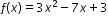and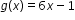can be expressed as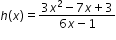, where the domain of h(x) is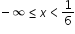and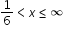.
MA.912.FL.3.2: Solve real-world problems involving simple, compound and continuously compounded interest.
 Clarifications:Clarification 1: Within the Algebra 1 course, interest is limited to simple and compound.

 Examples:Example: Find the amount of money on deposit at the end of 5 years if you started with \$500 and it was compounded quarterly at 6% interest per year. Example: Joe won \$25,000 on a lottery scratch-off ticket. How many years will it take at 6% interest compounded yearly for his money to double?
MA.912.FL.3.4: Explain the relationship between simple interest and linear growth. Explain the relationship between compound interest and exponential growth and the relationship between continuously compounded interest and exponential growth.
 Clarifications:Clarification 1: Within the Algebra 1 course, exponential growth is limited to compound interest.
MA.912.NSO.1.1: Extend previous understanding of the Laws of Exponents to include rational exponents. Apply the Laws of Exponents to evaluate numerical expressions and generate equivalent numerical expressions involving rational exponents.
 Clarifications:Clarification 1: Instruction includes the use of technology when appropriate. Clarification 2: Refer to the K-12 Formulas (Appendix E) for the Laws of Exponents. Clarification 3: Instruction includes converting between expressions involving rational exponents and expressions involving radicals.Clarification 4:Within the Mathematics for Data and Financial Literacy course, it is not the expectation to generate equivalent numerical expressions.
MA.912.NSO.1.2: Generate equivalent algebraic expressions using the properties of exponents.
 Examples:The expression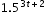is equivalent to the expression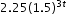which is equivalent to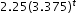.
MA.912.NSO.1.4: Apply previous understanding of operations with rational numbers to add, subtract, multiply and divide numerical radicals.
 Clarifications:Clarification 1: Within the Algebra 1 course, expressions are limited to a single arithmetic operation involving two square roots or two cube roots.

 Examples:Algebra 1 Example: The expression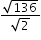is equivalent to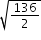which is equivalent to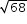which is equivalent to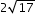.
MA.K12.MTR.1.1: Actively participate in effortful learning both individually and collectively.

Mathematicians who participate in effortful learning both individually and with others:

• Analyze the problem in a way that makes sense given the task.
• Build perseverance by modifying methods as needed while solving a challenging task.
• Stay engaged and maintain a positive mindset when working to solve tasks.
• Help and support each other when attempting a new method or approach.

 Clarifications:Teachers who encourage students to participate actively in effortful learning both individually and with others: Cultivate a community of growth mindset learners.  Foster perseverance in students by choosing tasks that are challenging. Develop students’ ability to analyze and problem solve. Recognize students’ effort when solving challenging problems.
MA.K12.MTR.2.1: Demonstrate understanding by representing problems in multiple ways.

Mathematicians who demonstrate understanding by representing problems in multiple ways:

• Build understanding through modeling and using manipulatives.
• Represent solutions to problems in multiple ways using objects, drawings, tables, graphs and equations.
• Progress from modeling problems with objects and drawings to using algorithms and equations.
• Express connections between concepts and representations.
• Choose a representation based on the given context or purpose.
 Clarifications:Teachers who encourage students to demonstrate understanding by representing problems in multiple ways: Help students make connections between concepts and representations.Provide opportunities for students to use manipulatives when investigating concepts.Guide students from concrete to pictorial to abstract representations as understanding progresses.Show students that various representations can have different purposes and can be useful in different situations.
MA.K12.MTR.3.1: Complete tasks with mathematical fluency.

Mathematicians who complete tasks with mathematical fluency:

• Select efficient and appropriate methods for solving problems within the given context.
• Maintain flexibility and accuracy while performing procedures and mental calculations.
• Complete tasks accurately and with confidence.
• Adapt procedures to apply them to a new context.
• Use feedback to improve efficiency when performing calculations.
 Clarifications:Teachers who encourage students to complete tasks with mathematical fluency:Provide students with the flexibility to solve problems by selecting a procedure that allows them to solve efficiently and accurately.Offer multiple opportunities for students to practice efficient and generalizable methods.Provide opportunities for students to reflect on the method they used and determine if a more efficient method could have been used.
MA.K12.MTR.4.1: Engage in discussions that reflect on the mathematical thinking of self and others.

Mathematicians who engage in discussions that reflect on the mathematical thinking of self and others:

• Communicate mathematical ideas, vocabulary and methods effectively.
• Analyze the mathematical thinking of others.
• Compare the efficiency of a method to those expressed by others.
• Recognize errors and suggest how to correctly solve the task.
• Justify results by explaining methods and processes.
• Construct possible arguments based on evidence.
 Clarifications:Teachers who encourage students to engage in discussions that reflect on the mathematical thinking of self and others:Establish a culture in which students ask questions of the teacher and their peers, and error is an opportunity for learning.Create opportunities for students to discuss their thinking with peers.Select, sequence and present student work to advance and deepen understanding of correct and increasingly efficient methods.Develop students’ ability to justify methods and compare their responses to the responses of their peers.
MA.K12.MTR.5.1: Use patterns and structure to help understand and connect mathematical concepts.

Mathematicians who use patterns and structure to help understand and connect mathematical concepts:

• Focus on relevant details within a problem.
• Create plans and procedures to logically order events, steps or ideas to solve problems.
• Decompose a complex problem into manageable parts.
• Relate previously learned concepts to new concepts.
• Look for similarities among problems.
• Connect solutions of problems to more complicated large-scale situations.
 Clarifications:Teachers who encourage students to use patterns and structure to help understand and connect mathematical concepts:Help students recognize the patterns in the world around them and connect these patterns to mathematical concepts.Support students to develop generalizations based on the similarities found among problems.Provide opportunities for students to create plans and procedures to solve problems.Develop students’ ability to construct relationships between their current understanding and more sophisticated ways of thinking.
MA.K12.MTR.6.1: Assess the reasonableness of solutions.

Mathematicians who assess the reasonableness of solutions:

• Estimate to discover possible solutions.
• Use benchmark quantities to determine if a solution makes sense.
• Check calculations when solving problems.
• Verify possible solutions by explaining the methods used.
• Evaluate results based on the given context.
 Clarifications:Teachers who encourage students to assess the reasonableness of solutions:Have students estimate or predict solutions prior to solving.Prompt students to continually ask, “Does this solution make sense? How do you know?”Reinforce that students check their work as they progress within and after a task.Strengthen students’ ability to verify solutions through justifications.
MA.K12.MTR.7.1: Apply mathematics to real-world contexts.

Mathematicians who apply mathematics to real-world contexts:

• Connect mathematical concepts to everyday experiences.
• Use models and methods to understand, represent and solve problems.
• Perform investigations to gather data or determine if a method is appropriate. • Redesign models and methods to improve accuracy or efficiency.
 Clarifications:Teachers who encourage students to apply mathematics to real-world contexts:Provide opportunities for students to create models, both concrete and abstract, and perform investigations.Challenge students to question the accuracy of their models and methods.Support students as they validate conclusions by comparing them to the given situation.Indicate how various concepts can be applied to other disciplines.
ELA.K12.EE.1.1: Cite evidence to explain and justify reasoning.
 Clarifications:K-1 Students include textual evidence in their oral communication with guidance and support from adults. The evidence can consist of details from the text without naming the text. During 1st grade, students learn how to incorporate the evidence in their writing.2-3 Students include relevant textual evidence in their written and oral communication. Students should name the text when they refer to it. In 3rd grade, students should use a combination of direct and indirect citations.4-5 Students continue with previous skills and reference comments made by speakers and peers. Students cite texts that they’ve directly quoted, paraphrased, or used for information. When writing, students will use the form of citation dictated by the instructor or the style guide referenced by the instructor. 6-8 Students continue with previous skills and use a style guide to create a proper citation.9-12 Students continue with previous skills and should be aware of existing style guides and the ways in which they differ.
 Clarifications:See Text Complexity for grade-level complexity bands and a text complexity rubric.
ELA.K12.EE.3.1: Make inferences to support comprehension.
 Clarifications:Students will make inferences before the words infer or inference are introduced. Kindergarten students will answer questions like “Why is the girl smiling?” or make predictions about what will happen based on the title page. Students will use the terms and apply them in 2nd grade and beyond.
ELA.K12.EE.4.1: Use appropriate collaborative techniques and active listening skills when engaging in discussions in a variety of situations.
 Clarifications:In kindergarten, students learn to listen to one another respectfully.In grades 1-2, students build upon these skills by justifying what they are thinking. For example: “I think ________ because _______.” The collaborative conversations are becoming academic conversations.In grades 3-12, students engage in academic conversations discussing claims and justifying their reasoning, refining and applying skills. Students build on ideas, propel the conversation, and support claims and counterclaims with evidence.
ELA.K12.EE.5.1: Use the accepted rules governing a specific format to create quality work.
 Clarifications:Students will incorporate skills learned into work products to produce quality work. For students to incorporate these skills appropriately, they must receive instruction. A 3rd grade student creating a poster board display must have instruction in how to effectively present information to do quality work.
ELA.K12.EE.6.1: Use appropriate voice and tone when speaking or writing.
 Clarifications:In kindergarten and 1st grade, students learn the difference between formal and informal language. For example, the way we talk to our friends differs from the way we speak to adults. In 2nd grade and beyond, students practice appropriate social and academic language to discuss texts.
ELD.K12.ELL.MA.1: English language learners communicate information, ideas and concepts necessary for academic success in the content area of Mathematics.

## General Course Information and Notes

### VERSION DESCRIPTION

In Algebra 1 Honors, instructional time will emphasize five areas: (1) performing operations with polynomials and radicals, and extending the Laws of Exponents to include rational exponents; (2) extending understanding of functions to linear, quadratic and exponential functions and using them to model and analyze real-world relationships; (3) solving quadratic equations in one variable and systems of linear equations and inequalities in two variables; (4) building functions, identifying their key features and representing them in various ways and (5) representing and interpreting categorical and numerical data with one and two variables.

All clarifications stated, whether general or specific to Algebra I Honors, are expectations for instruction of that benchmark.

Curricular content for all subjects must integrate critical-thinking, problem-solving, and workforce-literacy skills; communication, reading, and writing skills; mathematics skills; collaboration skills; contextual and applied-learning skills; technology-literacy skills; information and media-literacy skills; and civic-engagement skills.

### GENERAL NOTES

Honors and Accelerated Level Course Note: Accelerated courses require a greater demand on students through increased academic rigor.  Academic rigor is obtained through the application, analysis, evaluation, and creation of complex ideas that are often abstract and multi-faceted.  Students are challenged to think and collaborate critically on the content they are learning. Honors level rigor will be achieved by increasing text complexity through text selection, focus on high-level qualitative measures, and complexity of task. Instruction will be structured to give students a deeper understanding of conceptual themes and organization within and across disciplines. Academic rigor is more than simply assigning to students a greater quantity of work.

Florida’s Benchmarks for Excellent Student Thinking (B.E.S.T.) Standards
This course includes Florida’s B.E.S.T. ELA Expectations (EE) and Mathematical Thinking and Reasoning Standards (MTRs) for students. Florida educators should intentionally embed these standards within the content and their instruction as applicable. For guidance on the implementation of the EEs and MTRs, please visit https://www.cpalms.org/Standards/BEST_Standards.aspx and select the appropriate B.E.S.T. Standards package.

English Language Development ELD Standards Special Notes Section:

Teachers are required to provide listening, speaking, reading and writing instruction that allows English language learners (ELL) to communicate information, ideas and concepts for academic success in the content area of Mathematics. For the given level of English language proficiency and with visual, graphic, or interactive support, students will interact with grade level words, expressions, sentences and discourse to process or produce language necessary for academic success. The ELD standard should specify a relevant content area concept or topic of study chosen by curriculum developers and teachers which maximizes an ELL’s need for communication and social skills. To access an ELL supporting document which delineates performance definitions and descriptors, please click on the following link: https://cpalmsmediaprod.blob.core.windows.net/uploads/docs/standards/eld/ma.pdf

### General Information

 Course Number: 1200320 Course Path: Section: Grades PreK to 12 Education Courses > Grade Group: Grades 9 to 12 and Adult Education Courses > Subject: Mathematics > SubSubject: Algebra > Abbreviated Title: ALG 1 HONORS Number of Credits: One (1) credit Course Attributes: Honors Class Size Core Required Florida Standards Course Course Type: Core Academic Course Course Level: 3 Course Status: State Board Approved Grade Level(s): 9,10,11,12 Graduation Requirement: Algebra 1

#### Equivalent Courses

 1200310-Algebra 1Equivalency start year: 2014 1200386-Pre-Advanced Placement Algebra 1Equivalency start year: 2018

 Florida Algebra I HonorsAgile Mind, the Charles A. Dana Center at the University of Texas at Austin - Agile Mind Educational Holdings, Inc. - 2021 - 2021Math Nation: Florida's B.E.S.T. Algebra 1 HonorsMath Nation - Math Nation (a division of Study Edge) - 1 - 2023